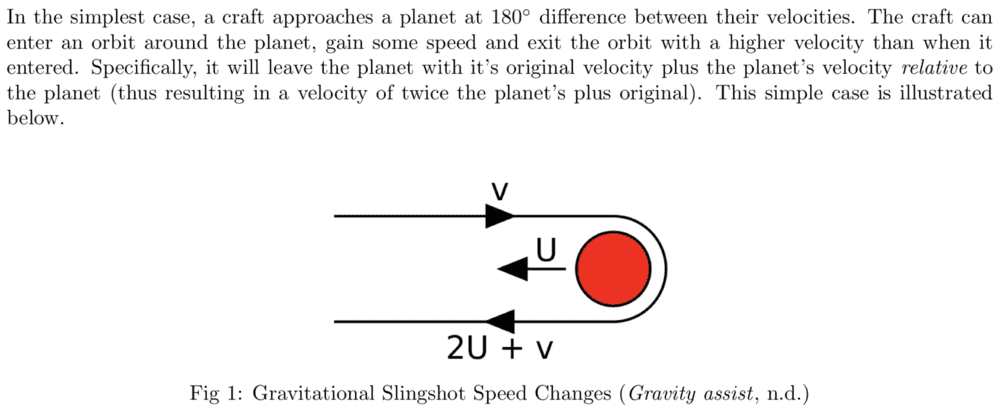# One dimensional elastic collision

pixel
A simple model often used to explain solar system gravitational slingshots is to consider a mass moving to the right with initial velocity v1i and a much larger mass moving to the left with initial velocity v2i. After the collision, the first mass is moving to the left with velocity v1f and the larger mass continues to move to the left with velocity v2f. As vrelative,before = -vrelative,after we can write (assuming all v's are positive quantities):

v1i + v2i = - (-v1f + v2f)​

v1f = v1i + v2i + v2f
It's common to say that since the planet (our large mass here) is so big it's velocity doesn't change much, so it's a good approximation to equate its initial and final velocities, leading to

v1f = v1i + 2v2i
But the smaller mass gets its velocity boost by "stealing" some from the larger mass. If we impose the condition that the initial and final velocities of the larger mass are exactly equal, why doesn't the equation show that the boost effect is eliminated?

In further considering this, I think forcing the signs of the final velocities prejudices the calculation. Obviously, if you set the larger mass' initial and final velocities to be equal in the momentum conservation equation, then the smaller mass' initial and final velocities would also be equal, i.e. no collision.

Last edited:

Homework Helper
As vrelative,before = -vrelative,after we can write (assuming all v's are positive quantities):
Why would that be the case? If there is gravitational attraction, there is an interaction over distance so the relative speeds will be constantly changing. I am also unclear how this can be a gravity assist slingshot and be a one dimensional collision.

AM

Mentor
But the smaller mass gets its velocity boost by "stealing" some from the larger mass. If we impose the condition that the initial and final velocities of the larger mass are exactly equal, why doesn't the equation show that the boost effect is eliminated?
Try working out the exact solution using conservation of momentum and kinetic energy: you’ll get two equations which can be solved to give you formulas for the two unknowns, the final speed of each mass. Now if you look at these two formulas you’ll see how a negligible change in the speed of the heavier mass can lead to a non-negligible change in the speed of the smaller one.

pixel
Try working out the exact solution using conservation of momentum and kinetic energy: you’ll get two equations which can be solved to give you formulas for the two unknowns, the final speed of each mass. Now if you look at these two formulas you’ll see how a negligible change in the speed of the heavier mass can lead to a non-negligible change in the speed of the smaller one.

Yes. I realized that as soon as I posted my question, hence the last paragraph that I added in edit.

pixel
Why would that be the case? If there is gravitational attraction, there is an interaction over distance so the relative speeds will be constantly changing.

In the non-gravitational model I presented, the speeds are constant before and after the collision. In the case of a gravitational slingshot, the "initial" and "final" speeds are considered to be before and after the interaction (collision) so that they are again constant.

I am also unclear how this can be a gravity assist slingshot and be a one dimensional collision.

It's just a one-dimensional example of a slingshot (from Orbital Mechanics of Gravitational Slingshots):Homework Helper
Orbital Mechanics of Gravitational Slingshots said:
In the simplest case, a craft approaches a planet at 180◦ difference between their velocities. The craft can enter an orbit around the planet, gain some speed and exit the orbit with a higher velocity than when it entered. Specifically, it will leave the planet with it’s original velocity plus the planet’s velocity relative to the planet (thus resulting in a velocity of twice the planet’s plus original). This simple case is illustrated below.
This passage is both poorly written and erroneous.

"The planet's velocity relative to the planet" should read "the planets velocity relative to the chosen rest frame. The planet's velocity relative to itself is zero, of course.

It is rather pointless to apply such a qualifier to the planet's velocity when no similar qualifier was applied to the craft's velocity.

pixel
This passage is both poorly written and erroneous.

"The planet's velocity relative to the planet" should read "the planets velocity relative to the chosen rest frame. The planet's velocity relative to itself is zero, of course.

It is rather pointless to apply such a qualifier to the planet's velocity when no similar qualifier was applied to the craft's velocity.

Maybe it could have been worded better, but in the context of the article I think it is clear that the craft's velocity and the planet's velocity are w.r.t. the rest frame. With that understanding, the craft does leave the planet with original velocity + planet's velocity relative to the planet, i.e. the relative velocity w.r.t. the planet. Then in the rest frame the craft has original velocity + 2 x the planet's velocity.